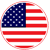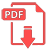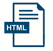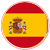# Home | Mathematics | Quadratic equation

## Algebra, Equation, Solving

When we simplify an expression we operate in the following order:

Simplify the expressions inside parentheses, brackets, braces and fractions bars.
1.Evaluate all powers.
2.Do all multiplications and division from left to right.
3.Do all addition and subtractions from left to right.

A useful rule is the denominator-numerator rule which states that the denominator and numerator may be multiplied by the same quantity without changing the value of the fraction.EnglishSpanish